#### Howdy, Stranger!

It looks like you're new here. Sign in or register to get started.

# Fitting cubic Bézier curves

The problem of curve fitting is fundamental to font technology, as we want to make Béziers which most closely resemble the "true" shape of the glyph. Font tools need to apply curve fitting to simplify outlines, apply transformations such as offset curve, delete a smooth on-curve point, and other applications. However, the problem is surprisingly tricky and there is no definitive solution in the literature. I recently worked out a much better solution and wrote about it in my blog:

https://raphlinus.github.io/curves/2021/03/11/bezier-fitting.html

The main reason it's so tricky is that C-shaped Bézier curves come in triplets of similar shape, causing local minima so that an optimizer may fall into one of these minima, even if one of the other two has lower error. My blog post gives a relatively simple formula for finding all three minima.

One subtle detail is that the goal of optimizing Bézier curves for shape accuracy is in tension with making them interpolation compatible. To me, this is a strike against cubic Béziers as a fundamental shape representation for fonts, and I continue to explore techniques that have smoothness guarantees by construction. But hopefully that's a topic for a future blog post.
Tagged:
«1

• edited March 2021
Just a while ago I have written a brute force patch for more accurate bézier merging in FontForge. I have thought for weeks of how I could make it faster... I considered using the area between the merged solution and the two original curves as a measure for the error (which of course is problematic due to the intersections which are difficult to calculate). Your idea of combining "integral between = 0" with a different measure (the x-moment) is very creative.
Thank you very much for the link to your blog and your precious research! I did not check yet your formulae by now but will look into them as soon as I have time and try them to implement for FontForge.
Your idea may also contribute to my FontForge plug-in "Curvatura", which has an algorithm that creates G2–continuity for cubic bézier curves by scaling the handles. The algorithm uses the energy \int κ^2(t) ds to determine which of the solutions of the quartic is the best, but the x-moment could be a faster replacement.

• Huge fan of your work on Spiro @Raph Levien and been following your blog for yrs. Very cool to see you in this forum.
• edited March 2021
@Raph Levien After reading your blog post more thoroughly I have a question and I hope you don't mind if I ask you for a hint here:
As far as I understand, the first moment is determined only for the approximation curve without any connection to the source curve (delta1 may depend on the area of the source curve, but delta0 is independent). How can it be a measure for the error then?
• You also measure the x-moment of the source curve. So the error is the difference between the x-moment of the source curve and the Bézier.

At some point I'll release code (still working on it). I could also polish up my Python notebook a bit and release that, but at present it's in a pretty messy state. In any case, I'd be thrilled to see it start to be applied in type tools.
• edited March 2021
@Raph Levien Thanks for your clarification!
Meanwhile, I have checked your formulae for the area and the moment of the normalized bezier curve. (I have used the formula for the signed area of a cubic bezier path a .. controls b and c .. d to the x-axis = ((xb-xa)*(10*ya+6*yb+3*yc+yd)+(xc-xb)*(4*ya+6*yb+6*yc+4*yd)+(xd-xc)*(ya+3*yb+6*yc+10*yd))/20)
I have got the same results for the area. For the first moment of the area I have got the same result for the first moment about the y-axis i.e. \int x dA = \int x dA/dt dt (the notation moment_x confused me a bit).
For the first moment of a cubic bezier path a .. controls b and c .. d (about the y-axis) I have got:
(280*xd^2*yd-105*xc*xd*yd-30*xb*xd*yd-5*xa*xd*yd-45*xc^2*yd-45*xb*xc*yd-12*xa*xc*yd-18*xb^2*yd-15*xa*xb*yd-5*xa^2*yd+105*xd^2*yc+45*xc*xd*yc-3*xa*xd*yc-27*xb*xc*yc-18*xa*xc*yc-27*xb^2*yc-45*xa*xb*yc-30*xa^2*yc+30*xd^2*yb+45*xc*xd*yb+18*xb*xd*yb+3*xa*xd*yb+27*xc^2*yb+27*xb*xc*yb-45*xa*xb*yb-105*xa^2*yb+5*xd^2*ya+15*xc*xd*ya+12*xb*xd*ya+5*xa*xd*ya+18*xc^2*ya+45*xb*xc*ya+30*xa*xc*ya+45*xb^2*ya+105*xa*xb*ya-280*xa^2*ya)/840
Did you receive the same result?
• That looks right, but I haven't looked in great detail. It's the moment along the x axis, which is the same as "about" the y axis. It's possible my terminology is nonstandard, but I think so. I might add that momentsPen in FontTools is an excellent existing implementation of the concept, and might be relevant to what you're doing.
• edited March 2021
@Raph Levien Your terminology is fine for me - I just wanted to be sure that we are speaking of the same thing. Meanwhile, I have implemented your algorithm in a proof-of-concept Python script. Additionally, from the quartic equation roots I have finally chosen the solution that is nearest to the other moment (\int y dA). As far as I have tested, the approximation works really well. E.g.: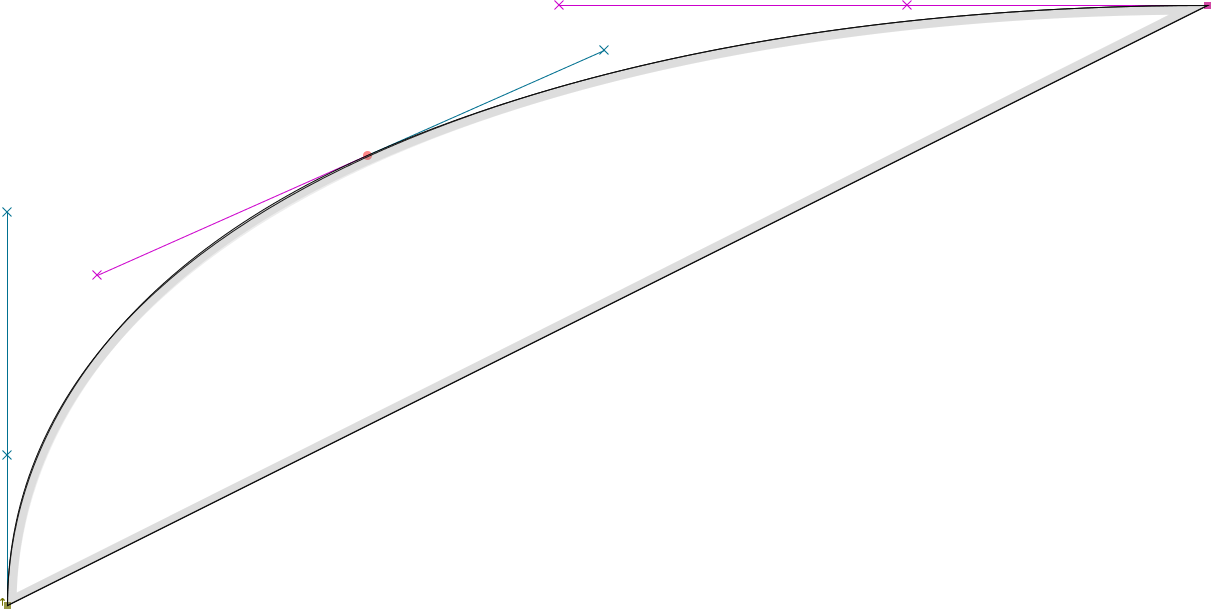There are surely some special cases that need to be handled carefully (chord length = 0, delta=90°) but I cannot see, why you have restricted your algorithm to C-shaped curves. Curves with inflections seem to work well, too: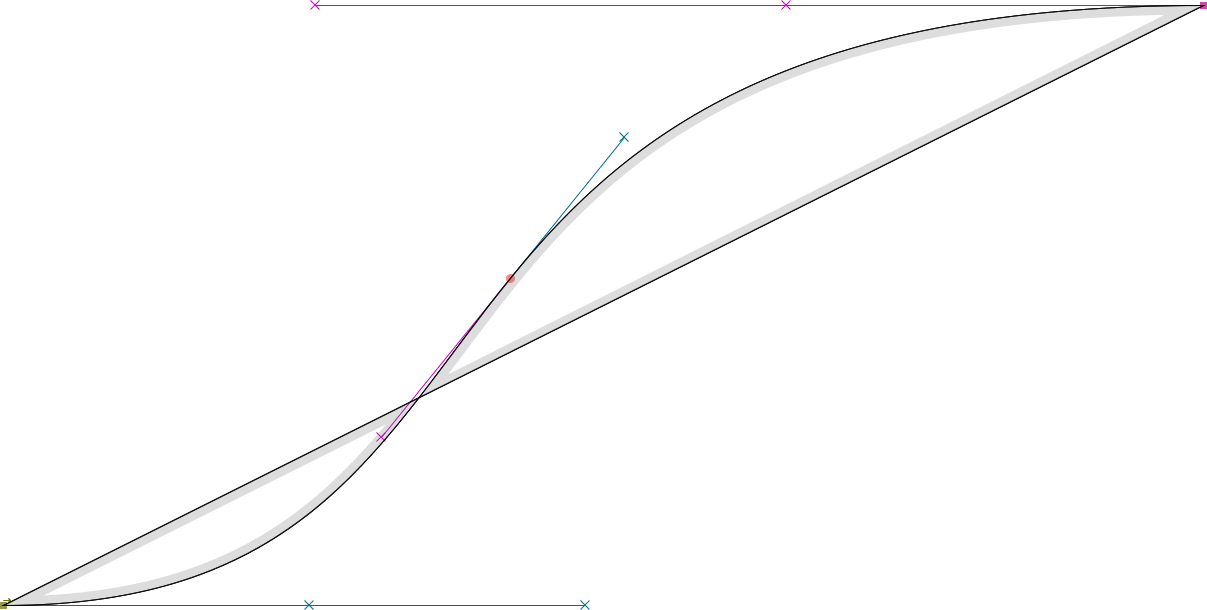• Excellent news, and it is true that chord length = 0 is a special case. But I haven't restricted it to C-shaped curves only, it's just those that have multiple solutionsChoosing the solution close to the other moment probably works well, but I haven't tested it carefully. In my notebook, and likely in the version I implement in kurbo, I'm computing an L2 error norm based on the normalized arc length parameterization of the two curves.
• Thank you, Raph, article is great as always!

Btw, while experimenting with the curve algorithms I found that a great way to visualize before/after difference is to make a closed path with the original and modified curve: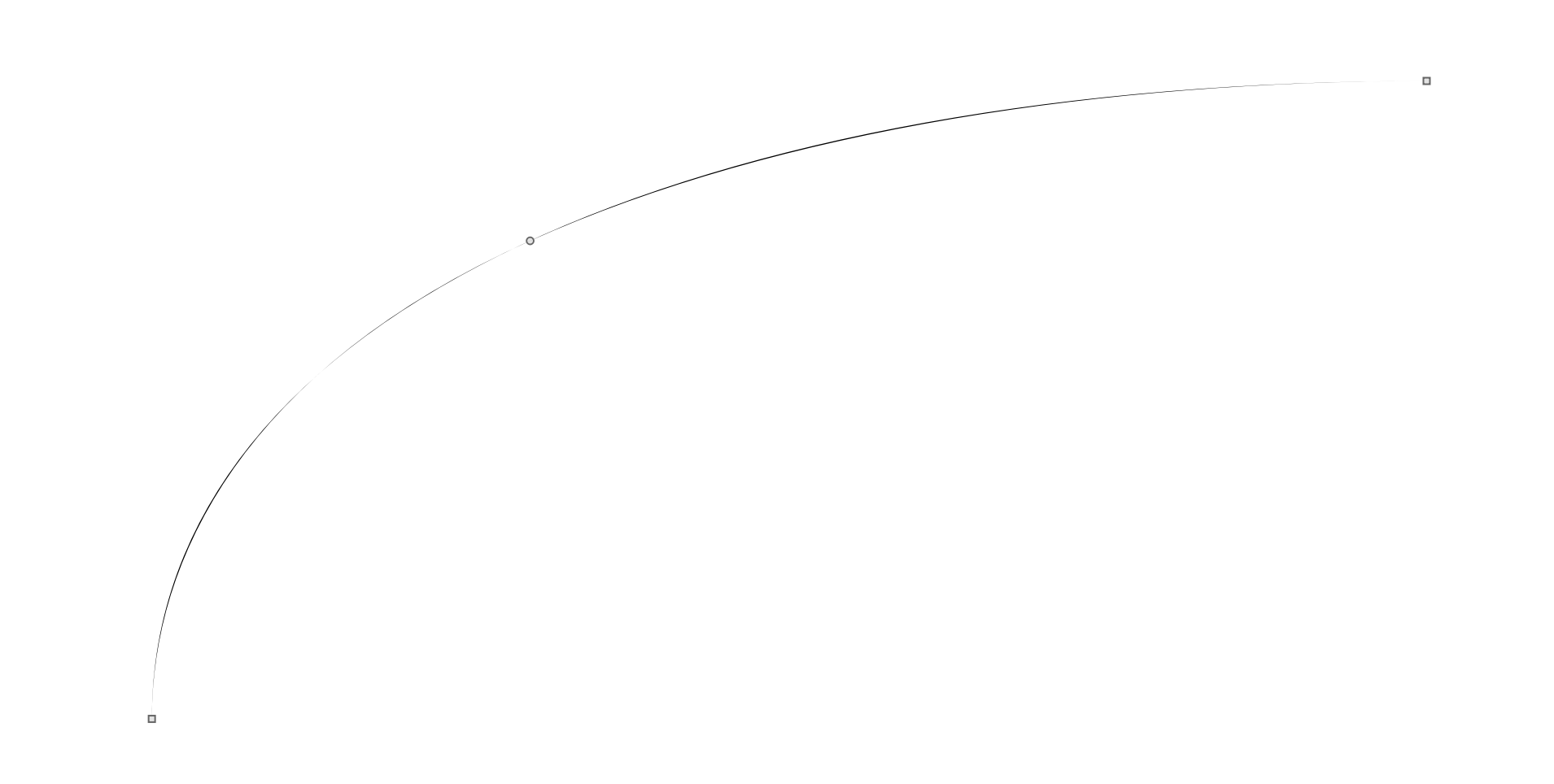• I found that a great way to visualize
Yes, very useful! Suggestion: use different colors depending on which curve is on which side.
• Hrant H. Papazian said:

Yes, very useful! Suggestion: use different colors depending on which curve is on which side.

That is possible to do with 2 overlapping colored contours one of which is semi-transparent. Can be done in modern tools, but the b/w option is much easier to do (duplicate segment, drag back to auto-join at the ends).
• To me, this is a strike against cubic Béziers as a fundamental shape representation for fonts, and I continue to explore techniques that have smoothness guarantees by construction.
Does this mean that VF-friendly outline simplification would work better in TTF?
Ever since I learned fontcrunch won’t help make VFs smaller (thank you, it helped me save ~10% on font weight!), I’ve been fantasising about progressive glyphs fidelity enhancement for VF fonts on the web where the data would first deliver a close but approximate visual match for body-size runs and only later bring in all the fine details visible at larger sizes (and masters/instances not visible in the first view).
• @Raph Levien I have now implemented your approximation in FontForge. Did you test half-circle-like beziers? When I tested the approximation of the two adjacent segments (100,0),(100,50),(50,100),(0,100) and (100,0),(-50,100),(-100,50),(-100,0), I found the approximation being slightly asymmetric: (-100,0),(-100,126.111111111),(100,132.555555556),(100,0)
The way the quartic polynomial is computed is probably crucial... I have used

[-9*ca*ca*(((2*sb*cb*ca+sa*(2*cb*cb-1))*ca-2*sb*cb)*ca-cb*cb*sa) ,
12*ca*((((cb*(30*f*cb-sb)-15*f)*ca+2*sa-cb*sa*(cb+30*f*sb))*ca+cb*(sb-15*f*cb))*ca-sa*cb*cb),
12*ca*((((70*m+15*f)*sb*sb+cb*(9*sb-70*cb*m-5*cb*f))*ca-5*sa*sb*(3*sb-4*cb*(7*m+f)))*ca-cb*(9*sb-70*cb*m-5*cb*f)),
16*ca*(((12*sa-5*ca*(42*m-17*f))*sb-70*cb*(3*m-f)*sa-75*ca*cb*f*f)*sb-75*cb*cb*f*f*sa),
80*ca*sb*(42*sb*m-25*f*(sb-cb*f))]
for the generic case

[9*ca^4*sa,
12*ca^3*(2*sa-15*ca*f),
12*ca^2*(ca*(70*m+15*f)-15*sa),
16*ca*(12*sa-5*ca*(42*m-17*f)),
80*ca*(42*m-25*f)]
for theta1=90

where sa = sin(theta0), sb = sin(theta1), ca = cos(theta0), cb = cos(theta1), m = moment, f = area and the polynomial coefficients start with x^4. Would you mind sharing the quartic polynomial that you are using?
• Linus: I have to clean up my notebook more than a bit, so it will be a few days, but there's enough interest it makes sense to do that. Right now internally it's using numerical techniques to find the roots instead of determining coefficients of a polynomial and using a quartic solver. Part of the reason for that is that I have more confidence in determining the "near misses" from closest approach of the x-moment than from the quartic polynomial itself, but have not yet evaluated whether the difference is significant. That said, when I plugged in your polynomial it was consistent with my numerical results. My notebook is mostly fitting Euler spirals and not yet systems of two cubic beziers (I'm working on that in Rust separately) so can't directly test your example. I'm not seeing any anomalies, though, when fitting an exact half circle.

So after I either clean up my Python notebook so I can release it, or get far enough into my Rust implementation to try your two-bezier input, I'd be able to compare more directly. In the meantime, I would be slightly suspicious that the area and moment of your input are exactly correct, as the asymmetry in output resembles what would happen if that were the case. In particular, if the input curve is symmetrical, the x-moment should be half the area; when I perturb the area slightly but change x-moment to match, both my numerical solver and your quartic agree on the result, which is symmetrical.

Mateusz: that's a very complex question. The previous blog post had an O(n^5) fit from Euler spirals (not based on moments or areas) that is not a problem for interpolation compatibility, but I think an O(n^6) solution will inevitably hit different branches.
• @Linus Romer Playing with this some more, I think the problem is numerical issues when theta0 is near 90 degrees, so ca approaches 0. Looking at your formulas, all coefficients have a ca term in them, so I believe it's safe and effective to simply take that out. Can you try that? You also have to make sure your solver is robust when the x^4 term approaches 0 - not all are.
• @Raph Levien Stupid me! It's actually obvious that ca is in all coefficients but I didn't see it. Thanks for your hint, it solved the asymmetry problem perfectly!
• @Linus Romer Yes, I'm sure the readers of this forum are all saying, "I don't see how Linus could have missed that obvious minor refactor to improve numerical stability after doing a perfectly straightforward derivation of the coefficients of the polynomial. I certainly wouldn't have made that mistake."

More seriously, I'm pleased and impressed you were able to get this to work. Your derivation is better than the direction I was headed - I was dividing by sin(θ₀+θ₁) to resolve the δ₀δ₁ terms, and was planning to special-case the case where that approaches zero. That seems to cancel out, and your coefficients seem pretty solid.

As I mentioned, the remaining case that I'm finding tricky is the "near misses." What's really bothering me there is that if we compute the roots of the derivative of that polynomial (ie solve the resulting cubic) we should get pretty good results, but it's not symmetric to a left-right swap. This is why I'm still leaning to numerically finding the zero-crossing of the derivative of the x-moment. I can think of a couple ways to do that, but haven't yet settled on one.

This feels like a real open source collaboration!
• edited March 2021
@Raph Levien Yes, your algorithm is not symmetric to a left-right swap. But I cannot see how this is connected to the way the roots of the derivative of the x-moment are computed. I think this asymmetry is inherent to your algorithm. And it is not that problematic: Can't one just consider both the original and the swapped version and take the solution with the lowest l2-error? (By the way, I am using an l2 error now as well to choose the best solution.)
I had some trouble with FontForge because the already built-in quartic solver is not as solid as I wished. Hence, I have written a simple Newton solver combined with polynomial divisions to get all roots. While testing I have made three interesting observations:
1. One should norm θ₀ to being non-negative (what you may have done anyway but I did not see any mathematical necessity). The numerical computations unfortunately seem to depend on the signs...
2. The case θ₁ = 180° - θ₀ leads to bad approximations and should be handled separately. This case happens quite often in type design: Think of the outer outline of an /o that consists of two horizontal and two vertical on-curve points. (In the end one probably want to add extrema again.)
3. There are situations (e.g. happening on the /o of the bold Euler Fraktur eufb10.pfb) where both the zeroes of the x-moment and the zeroes of its derivative are suboptimal (because δ1 is negative for them)! E.g. the x-moment has the function f(x):=0.113110x^4-0.334960x^3+0.302089x^2-0.140921x+0.048084(where x stands for δ0) and δ1(x)=(20*0.024743-6*x*sin(0.116270))/(3*(2*sin(0.220310)-x*sin(0.116270+0.220310))) and it looks like this (green = x-moment, orange = δ1):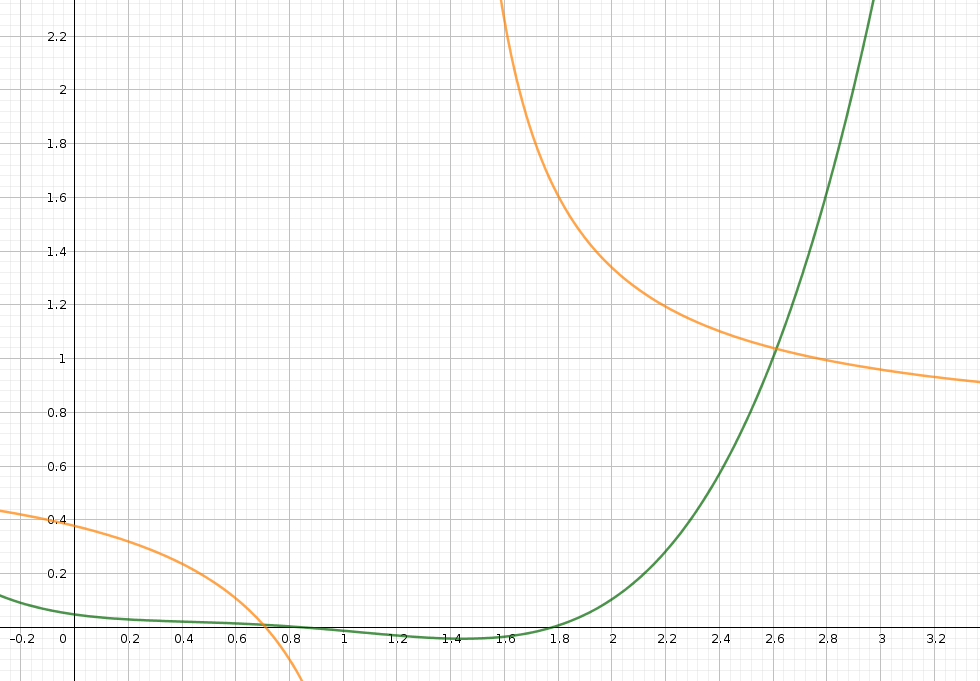I have solved the 3. problem by additionally considering cases where δ1 is approximately 0 (exactely 0 does not preserve the direction, I took 0.01 instead).

• I use your algorithm for "simplify" in Fontforge as well. Here is an example for the /S in the Castoro typeface: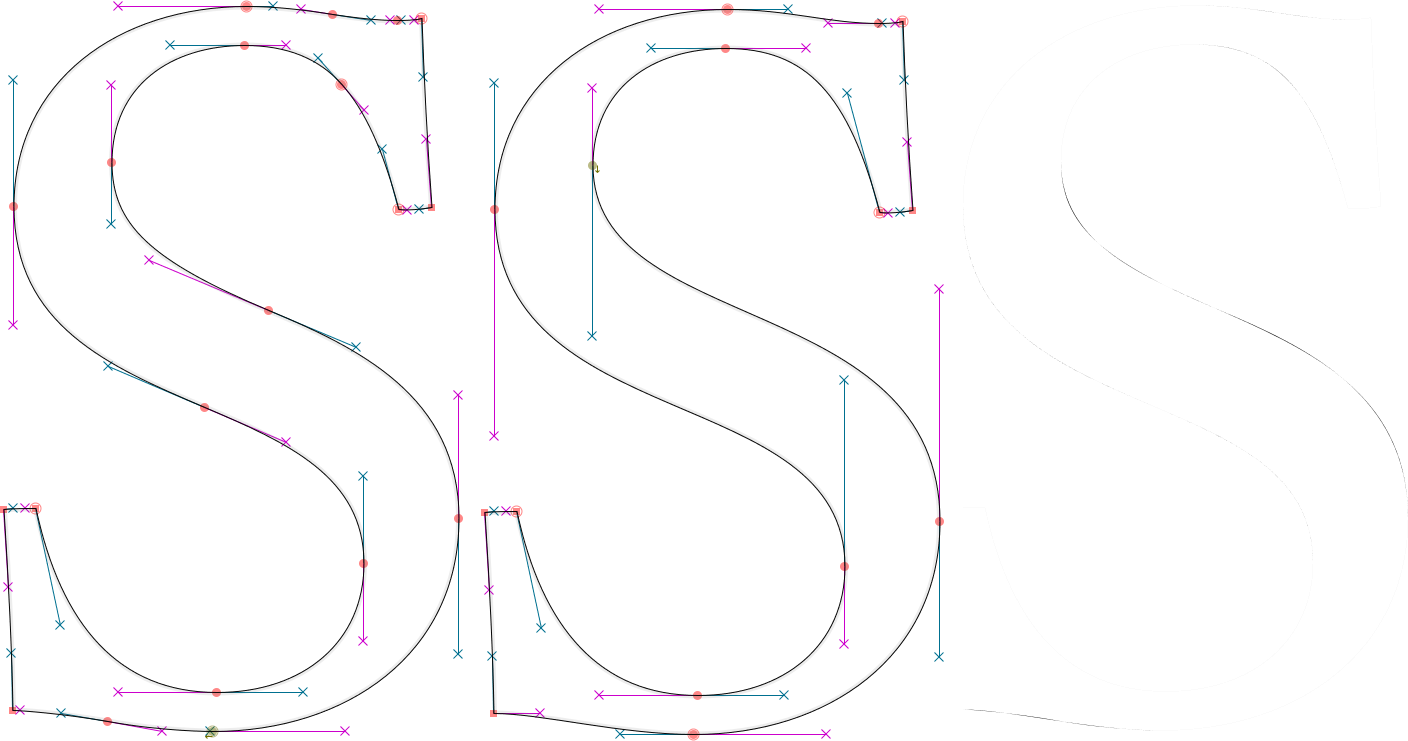• I use your algorithm for "simplify" in Fontforge as well. Here is an example for the /S in the Castoro typeface:

Looks impressive. ~45/60 reduction in points. If you count the pixels in the 3rd, rightmost image, you get the difference of the areas. Can also be done by xor of the two rendered images. Would be interesting how large the relative area-difference and the relative thickness difference is (pixel_difference / em_size).

In theory 1 unit / 2000 M-size = 0.0005 is smaller than visual resolution 0.15 mm / 250 mm reading distance = 0.0006.
• edited March 2021
That minor difference you can seen between the Castoro S and the simplfied version: that’s the reason I put the points where I put them.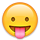• That minor difference you can seen between the Castoro S and the simplfied version: that’s the reason I put the points where I put them.Sure, as a fulltime typedrawer you have trained eyes (better: trained visual recognition). There are scientific papers showing evidence that trained vision can go to subpixel resolution ~2-fold. Also you have your methods for nitpicking.

The interesting part for me is a threshold, which we can use in software.

Left is Google rendered by Chrome right is Tiro rendered in Mac TextEdit. Look without cheating first at size 14 for the difference, then next sizes.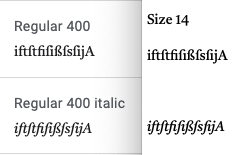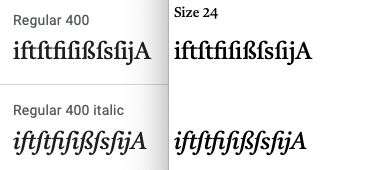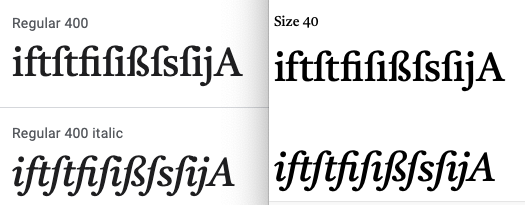For the readers not detecting it: The sequence longs_i is different between the two versions of the font.
• @Linus Romer Thanks for your feedback, there are some interesting points. A lot of what you say seems to be problems with the solver, not with the underlying ideas. In particular, the case of θ₀ + θ₁ =180° in my experiments yields a polynomial where the x^4 and x^3 coefficients are near-zero. As I warned upthread, that's a case where many solvers have trouble, as a standard technique is to normalize all the coefficients so that the highest order monomial has a coefficient of 1. This is one reasons I'm also balking at using a quartic root finder and tempted by numerical solving. But, as you're experiencing, getting that perfect is not trivial.

Newton solvers are tempting due to their high rate of convergence, and, in this case, ease of computing the derivative analytically. However, they go unstable when there are multiple roots (or nearly so), and that's also a case that can happen here. I've been exploring the ITP method and have it implemented in kurbo now, as it's extremely robust numerically as well as converging reasonably fast. I'm still chipping away at the kurbo implementation of curve fitting (I really want to get it perfect) and haven't made final decisions, but am leaning strongly toward ITP as the main solving technique.

Let me see if I can explain the left-right swap issue more clearly. If you're only looking at zeros of the x-moment error, those don't move if you multiply it by a factor of (aθ₀ + b)², which is what we do to get the quartic polynomial. Another way of phrasing this is that the x-moment error can be expressed as a rational polynomial with a quartic on top and that quadratic on bottom. And thus the zeros also don't move if you swap θ₀ and θ₁. But if you look at extrema of the x-moment error vs extrema of just the quartic (the latter of which is two different versions depending on whether it's expressed in terms of δ₀ or δ₁), all three are in different places. Yes, you could make it symmetrical by computing both of the latter extrema and choosing the minimal error, but don't you think it's more satisfying to pick the extremum of the x-moment itself?

Lastly, the question of solutions near δ = 0 is a tricky one, and I did gloss over it in my post. As you observe, the exact δ = 0 doesn't in general preserve G1 continuity. Alternatively, one could choose to preserve G1 at the cost of equal-area, by choosing the other control point to be exactly at the intersection of the tangents. Choosing a (somewhat arbitrary) small δ value, as you have done, preserves both G1 and area, but doesn't feel awesome to me, probably just because it's likely to produce a small region of very high curvature. I'm not 100% sure what the best thing to do is here, but your approach is certainly reasonable.

I should say: there are at least two applications I have in mind for this curve-fitting. One is simplification, of which merging two beziers into one is an even more specific case. In that case, the errors might be relatively large - easy enough to see by eye - and there are some judgment calls to be made. The other major case is rendering a curve to beziers where you have to meet some error threshold, as well as other constraints including G1 and equal area, but it's possible to use more beziers when needed. In that application domain, I think the best approach is to discard δ ≤ 0 solutions, and let the "outer loop" subdivide the curve into more beziers.

I'll update this thread when I make more progress on the "production" solver. In the meantime, your observations will no doubt be invaluable to anyone else trying to implement this.

• edited April 2021
That minor difference you can seen between the Castoro S and the simplfied version: that’s the reason I put the points where I put them.There's a much better reason than a minute difference in shape for marking explicit inflection points: converting to quadratic béziers (which can happen in software, without oversight) is far less likely to result in mangled contours.
• edited April 2021
In particular, the case of θ₀ + θ₁ =180° in my experiments yields a polynomial where the x^4 and x^3 coefficients are near-zero.
Indeed, for the exact cases θ₀ + θ₁ = 180° or θ₀ + θ₁ = 0° the first two coefficients are exactely 0 and the quartic becomes a quadratic equation.
Newton solvers are tempting due to their high rate of convergence, and, in this case, ease of computing the derivative analytically. However, they go unstable when there are multiple roots (or nearly so), and that's also a case that can happen here.
The newton solver seems to behave quite well in my experiments. I think the probability of a non-convergence is quite low in this application.
I've been exploring the ITP method and have it implemented in kurbo now, as it's extremely robust numerically as well as converging reasonably fast.
Isn't finding ya < 0 < yb quite difficult for a general quartic?
Let me see if I can explain the left-right swap issue more clearly. If you're only looking at zeros of the x-moment error, those don't move if you multiply it by a factor of (aθ₀ + b)², which is what we do to get the quartic polynomial. Another way of phrasing this is that the x-moment error can be expressed as a rational polynomial with a quartic on top and that quadratic on bottom. And thus the zeros also don't move if you swap θ₀ and θ₁. But if you look at extrema of the x-moment error vs extrema of just the quartic (the latter of which is two different versions depending on whether it's expressed in terms of δ₀ or δ₁), all three are in different places. Yes, you could make it symmetrical by computing both of the latter extrema and choosing the minimal error, but don't you think it's more satisfying to pick the extremum of the x-moment itself?

Thank you for pointing this out. Indeed, I have (falsely) assumed that the zeroes of the derivative of the quartic are the zeroes of the derivative of the moment. But of course you are right. However, the derivative of the moment ends up in a quartic as well.

The quartic for the roots of the derivative of the moment is:

0 = -9*ca*sa*sab^3 * a^4 -3*sab^2*(9*ca*sa*sb-(17*sa+30*ca*f)*sab+15*cb*sa^2) * a^3 +18*sab*sb*(21*ca*sa*sb-(17*sa+30*ca*f)*sab+15*cb*sa^2) * a^2 -4*(144*ca*sa*sb^3+((-78*sa-135*ca*f)*sab+108*cb*sa^2)*sb^2+(-125*f*sab^2-45*cb*f*sa*sab)*sb+150*cb*f^2*sab^2) * a +8*sb*((24*sa+45*ca*f)*sb^2+(15*cb*f*sa-125*f*sab)*sb+100*cb*f^2*sab)
where ca = cos(θ₀), sa = sin(θ₀), cb = cos(θ₁), sb = sin(θ₁), sab = sin(θ₀ + θ₁), f = area, a = δ₀.

This quartic equation reduces to a linear for the special cases θ₀ + θ₁ = 180° or θ₀ + θ₁ = 0°:

0 = -3*ca*sa * a + 4*sa+5*ca*f

When I check symmetry by just interchanging θ₀ and θ₁, the roots of the quartic of the moment-error are not symmetric (because the moment actually changes when θ₀ and θ₁ are interchanged), but the roots of the quartic of the derivative of the moment are symmetric (because the derivative of the moment is independent of the given moment). Here is an example:

θ₀ = 6.5°
θ₁ = 12.5°
area = 0.025
moment = 0.013
zeroes (θ₀,θ₁):
normal zero (-0.238080,0.432158)
normal zero (1.808710,1.556856)
derivative zero (0.381235,0.260242)
derivative zero (0.497571,0.199395)

θ₀ = 12.5°
θ₁ = 6.5°
area = 0.025
moment = 0.013
zeroes (θ₀,θ₁):
normal zero (0.501812,-0.802088)
normal zero (1.420217,1.899029)
derivative zero (0.260242,0.381235)
derivative zero (0.199395,0.497571)
• edited April 2021
That minor difference you can seen between the Castoro S and the simplfied version: that’s the reason I put the points where I put them.Of course! I have never intended to criticise Castoro.

I use your algorithm for "simplify" in Fontforge as well. Here is an example for the /S in the Castoro typeface:

I cannot understand how anybody could disagree with that... Let me assure that both statements are correct• @Raph Levien Upon your comment I have considered using a more stable newton solver (the two point newton method) but then I thoroughly tested the original quartic solver of FontForge, which is a mixture between a cubic solver and a binary search. The quartic solver of Fontforge proved to be stable and better than my newton solver (older tests had indicated the opposite but the tests must have been wrong). Hence, I have now ditched my newton solver in favour of the original solver.
• My pull request has been merged! This means the main branch of FontForge has @Raph Levien's algorithm implemented and the next release of FontForge will use it. (Of course, you can use it already if you compile the sources.)

• I'm coming back to this (sometimes it takes me a while, as I'm juggling so many things). I believe I now have a solution to both the asymmetry I was worrying about and the bad result in Euler Fraktur, as I believe they're actually the same thing. In this case there are still four roots of the quartic, two real and two complex (they are complex conjugates of each other). In this case, the best value is not the zero of the derivative of the moment, but rather the real part of the complex roots, which gives δ0 = 0.1677 and δ1 = 0.3303, which I believe are good values. The attached plot shows the situation graphically: the quartic has been factored into two quadratics.

Regarding poor approximations when the sum of the angles is 180°, I think in this case the equation becomes quadratic and it's best to just set the x^3 and x^4 terms to zero rather than try to run them through a quartic solver with epsilon values - my current solver code also doesn't handle this well, though I'm looking at ways of making it more robust. Also I can heartily recommend https://cristiano-de-michele.netlify.app/publication/orellana-2020/orellana-2020.pdf as a good approach to quartic solving.

I will post another update and some code before too long. The main reason I'm looking at this now is that I think it will be the basis of a most excellent solution to stroke offset.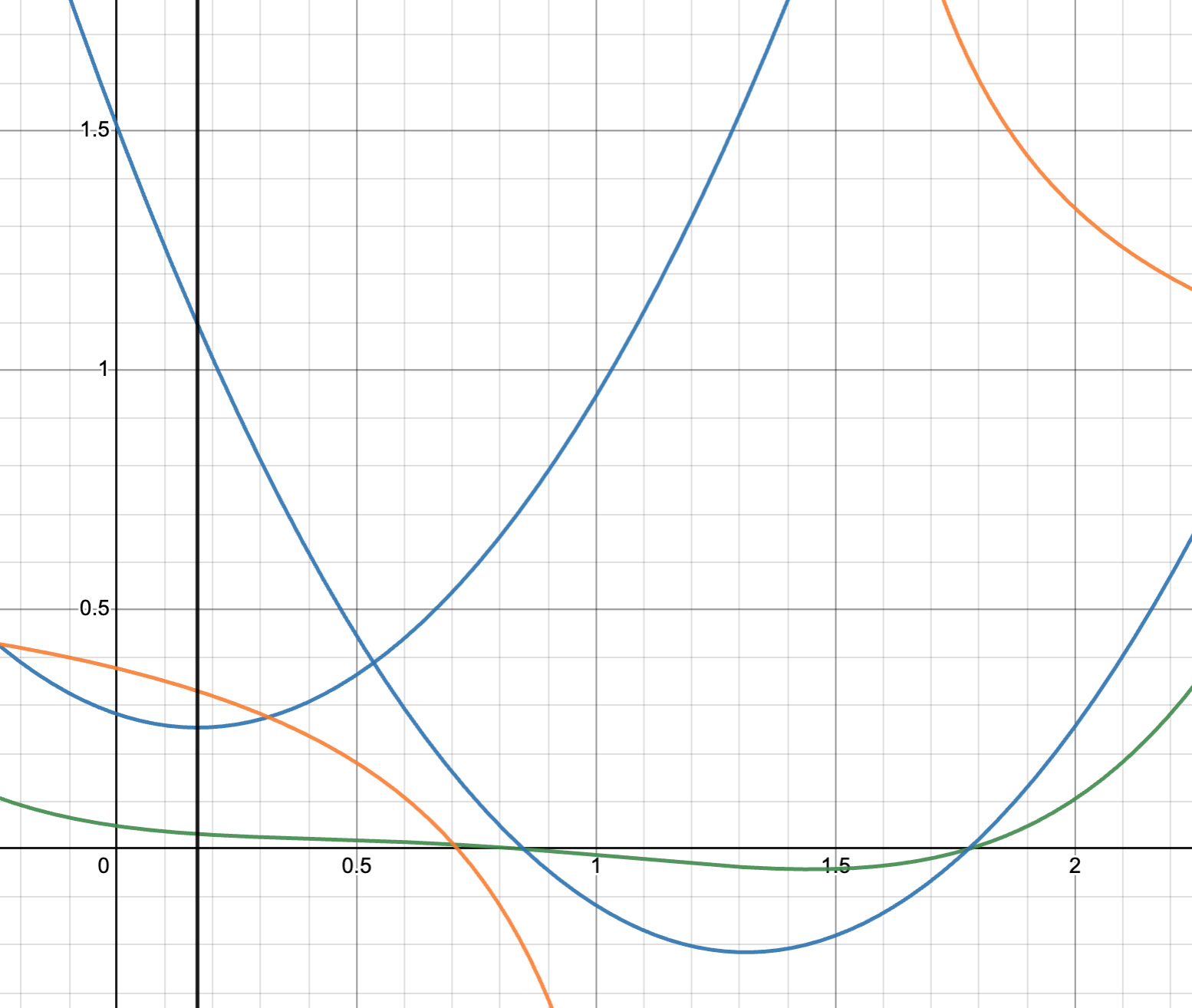• @Raph Levien Is there a mathematical reason for using the real part of the complex roots? For me it is not obvious to do so in this case.